##### Actions

(diff) ← Older revision | Latest revision (diff) | Newer revision → (diff)

An oriented link that has a diagram with all positive crossings (cf. also Link). More generally, a link is-almost positive if it has a diagram with all butof its crossings being positive.

The unknotting number (Gordian number) of a positive link is equal to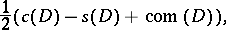whereis a positive diagram of the link,is the number of crossings,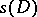is the number of Seifert circles of, and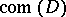is the number of components of the link (this generalizes the Milnor unknotting conjecture, 1969, and the Bennequin conjecture, 1981). Furthermore, for a positive knot the unknotting number is equal to the-ball genus of the knot, to the genus of the knot (cf. also Knot theory), to the planar genus of the knot (from the Seifert construction), to the minimal degree of the Jones polynomial, and to half the degree of the Alexander polynomial.

One can define a relation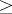on links by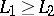if and only ifcan be obtained from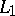by changing some positive crossings of. This relation allows one to express several fundamental properties of positive (and-almost positive) links:

1) Ifis a positive knot, then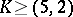positive torus knot unlessis a connected sum of pretzel knots, where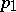,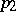andare positive odd numbers;

a) ifis a non-trivial positive knot, then either the signature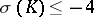oris a pretzel knot(and then);

b) if a positive knot has unknotting number one, then it is a positive twist knot.

2) Letbe a non-trivial-almost positive link. Then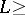right-handed trefoil knot (plus trivial components), orright-handed Hopf link (plus trivial components). In particular,has a negative signature.

3) Ifis a-almost positive knot, then either

i)right handed trefoil; or

ii)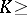mirror image of the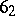-knot (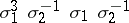in the braid notation); or

iii)is a twist knot with a negative clasp.

4) Ifis a-almost positive knot different from a twist knot with a negative clasp, thenhas negative signature and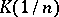(i.e.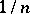surgery on,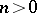; cf. also Surgery) is a homology-sphere that does not bound a compact, smooth homology-ball, [a1], [a6];

5) ifis a non-trivial-almost positive knot different from the Stevedore knot, thenis not a slice knot;

6) ifis a non-trivial-almost positive knot different from the figure eight knot, thenis not amphicheiral.

7) Letbe a-almost positive knot. Then eithertrivial knot oris the left-handed trefoil knot (plus positive knots as connected summands). In particular, eitherhas a non-positive signature oris the left-handed trefoil knot.

How to Cite This Entry: